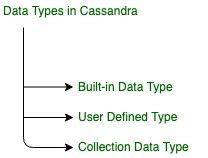GeeksforGeeks App
Open AppBrowser
Continue

# Pre-defined data type in Apache Cassandra

Prerequisite – User Defined Type (UDT) in Cassandra
In this article, we will discuss different types of data types in Cassandra which is used for various purpose in Cassandra such that in data modeling, to create a table, etc.

Basically, there are 3 types of data type in Cassandra. Lets have a look.Figure – Data Types in Cassandra

Now, here we are going to discuss the Built-in data type in Cassandra.

Built-in Data Type:
It is a pre-defined data type in Cassandra. We can directly use by simply giving data type names as per need. There are many Built-in Data types in Cassandra. let’s discuss one by one.

• Boolean:
It is a data type that represents two values, true or false. So, we can use such type of data type where we need just two values.

`true or false `

Syntax:

```CREATE TABLE table_name(
field_name1 Boolean,
...
); ```
• blob:
It is used for binary large objects such that audio, video or other multimedia and sometimes binary executable code stored as a blob.

`binary large objects `

Syntax:

```CREATE TABLE table_name(
field_name1 blob,
...
); ```
• ASCII:
It is used for strings type such that words and sentences. It represents the ASCII value of the character. For example, for ‘A’ ASCII value is 65. so, it will store its ASCII value.

`65 for A, 97 for a, etc. `

Syntax:

```CREATE TABLE table_name(
field_name1 ascii,
...
); ```
• bigint:
It is used for 64 bit signed long integer. Basically it is used for high range of integer which represents a value from -(2^32) to +(2^32) roughly.

`only for integers -(2^32) to +(2^32) `

Syntax:

```CREATE TABLE table_name(
field_name1 bigint,
...
); ```
• counter:
It is used for integers and represents a counter column. These columns part of the row which represents column family which basically contains numeric values, containing the number of columns.

`1, 2, 3... (integer)  `

Syntax:

```CREATE TABLE table_name(
field_name1 counter,
...
); ```
• Decimal:
It is used to save integer and float values. In Decimal data type is important to note when we tried to save decimal value such as .907 ( dot 907), it will give an error “no viable alternative at input ‘.’ (…DecimalValue) Values ( 1, [.]…)”. If we need to save decimal like that then start with zero e.g 0.907.

`10.45, 1, -1, 0.32...  `

Syntax:

```CREATE TABLE table_name(
field_name1 DECIMAL,
...
); ```
• Double:
It is used for integers which represents a 64 bit floating point. It include a number with a decimal points. for example: 5.838, 10.45 etc.

`10.4556, 3.566, 0.5875 etc.  `

Syntax:

```CREATE TABLE table_name(
field_name1 double,
...
); ```
• float:
It is used for numbers which represents a 32-bit floating point. It represents a number values with a decimal point. for example : 6.254, 5.23 etc.

`5.423, 2.31, 3.12...[32 bit floating point]  `

Syntax:

```CREATE TABLE table_name(
field_name1 float,
...
); ```
• inet:
It is used to represent an IP address that includes both numeric and characters. basically It Represents an IP address, IPv4 or IPv6. For example, 64.233.160.0 for such type address we can use inet data type.

`64.233.160.0 ...[IP address, IPv4 or IPv6]  `

Syntax:

```CREATE TABLE table_name(
field_name1 inet,
...
); ```
• int:
It is used to Represents 32-bit signed integers. It represents both positive and negative numbers. The range of int lies from -(2^16) to +(2^16) [only integers].

`24, 907, -9,  ... `

[Represents 32-bit signed int -(2^16) to +(2^16) [only integers]]

Syntax:

```CREATE TABLE table_name(
field_name1 int,
...
); ```
• text:
It is used to store string type which Represents UTF8 encoded string. It encodes all valid points in Uni-code by using 1 bit to four 8 bit types in Cassandra.

`Ashish, rana, ...[Represents UTF8 encoded string]   `

Syntax:

```CREATE TABLE table_name(
field_name1 text,
...
); ```
• varchar:
It is used to store arbitrary string which represents UTF8 encoded string.

`Ashish, rana, a\$#34, A67dgg...`

[arbitrary string, Represents UTF8 encoded string]

Syntax:

```CREATE TABLE table_name(
field_name1 varchar,
...
); ```
• timestamp:
It is used to represents a timestamp which is very helpful to store the exact time format value of timestamp. For example, if we want to store 15 dec 1995 at 4:00 AM then timestamp would be:

`1995-12-15 04:00 +0530 `

Here +0530 is represents meridian for India.

`[formats: yyyy-mm-dd HH:mm or yyyy-mm-dd HH:mm:ss]  `

Syntax:

```CREATE TABLE table_name(
field_name1 timestamp,
...
); ```
• variant:
It is used to represents arbitrary-precision integer such that 124, 24, 1, 5468 etc.

`1, 24, 07, 897, 4568, etc.  `

Syntax:

```CREATE TABLE table_name(
field_name1 variant,
...
); ```
My Personal Notes arrow_drop_up